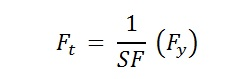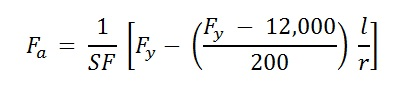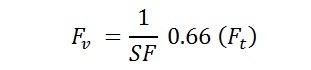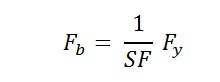Change List for this Rule

General Order 95

Section IV

Strength Requirements for All Classes of Lines

 48.2 Steel The required strength of steel structures and components shall be designed using ASCE 10 97 for latticed steel structures and ASCE 48 11 for tubular steel pole structures, as applicable. Allowable stresses for steel members and their connections shall be derived in conjunction with the safety factors in Rule 44 and the permitted stresses specified in the applicable standard. Steel members not covered by either of these standards shall be designed using allowable stresses as defined below: Tension: The maximum allowable tensile stress shall be calculated using the following formula:Compression: The maximum allowable compressive stress shall be calculated using the following formula:Shear: The maximum allowable shear stress shall be calculated using the following formula:Bending: The maximum allowable bending stress for a compact section shall be calculated using the following equation:The maximum allowable bending stress for a non-compact section shall be determined according to the provisions of Chapter E of the AISC Manual of Steel Construction, Allowable Stress Design, 9th Edition. Combined Stresses: The strength of members subjected to combined stresses shall be determined according to the provisions of Chapter H of the AISC Manual of Steel Construction, Allowable Stress Design, 9th Edition. Where, Fa = maximum allowable axial stress, psi Fb = maximum allowable bending stress, psi Ft = maximum allowable tensile stress, psi Fv = maximum allowable shear stress, psi Fy = specified minimum yield stress, psi Fu = specified minimum tensile stress, psi SF = safety factor as specified in Rule 44 l = unsupported length of member, inches r = radius of gyration of member, inches The values used for specified minimum yield stress, Fy, and specified minimum tensile stress, Fu, shall be the values as listed in the appropriate ASTM specification. If the material specification for the steel is unknown and cannot be determined, the values for Fy and Fu and shall be assumed to be 33,000 psi and 60,000 psi, respectively. The modulus of elasticity, E, is defined to be 29,000 ksi.